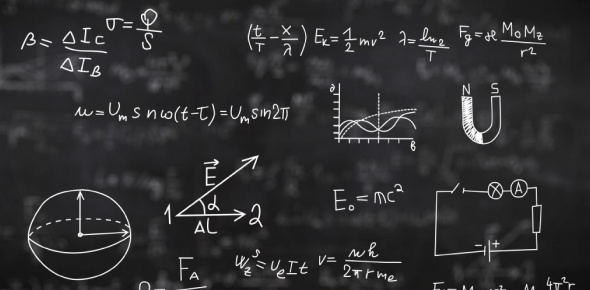# Physical Science Module 1

10 Questions | Total Attempts: 445SettingsCreate your own QuizThis quiz may be taken as many times as you wish as practice to increase comprehension of material. Have fun!

• 1.
What is the smallest chemical unit of matter?
• A.

Molecule

• B.

Atom

• C.

Solution

• D.

Concentration

• 2.
What are two or more atoms linked together to make a substance with unique properties?
• A.

Molecule

• B.

Atom

• C.

Solution

• D.

Concentration

• 3.
What is the quantity of a substance within a certain volume?
• A.

Atom

• B.

Molecule

• C.

Solution

• D.

Concentration

• 4.
Sulfur is a yellow powder that is composed of sulfur atoms.  Sulfur dioxide is a colorles, poisonous gas that contains sulfur atoms.  Is sulfur dioxide composd of atoms or molecules?
• A.

Atoms

• B.

Molecules

• 5.
You are reading a scientist's notes and you notice a measurement that is listed at "12.3 kilograms."  This measurement represents ____________________.
• A.

Length

• B.

Mass

• C.

Volume

• 6.
What metric prefix means "1000?"
• A.

Centi

• B.

Kilo

• C.

Milli

• D.

Deci

• 7.
How many centimeters are in 1.6 meters?
• A.

0.16 cm

• B.

1.6 cm

• C.

160 cm

• D.

1600 cm

• 8.
An object's volume is 0.12 kL.  What is its volume in liters?
• A.

0.12 L

• B.

1.2 L

• C.

12 L

• D.

120 L

• 9.
A rock has a mass of 45.1 kg.  What is its mass in slugs?  (1 slug = 14.49 kg)  Round to the nearest whole number.
• A.

3 kg

• B.

15 kg

• C.

45 kg

• D.

675 kg

• 10.
The average length of a cubit is ______________.
• A.

45 mm

• B.

45 cm

• C.

45 in

• D.

45 ft

Related TopicsBack to top
×

Wait!
Here's an interesting quiz for you.